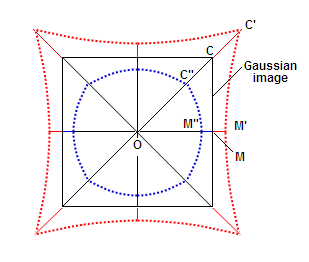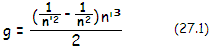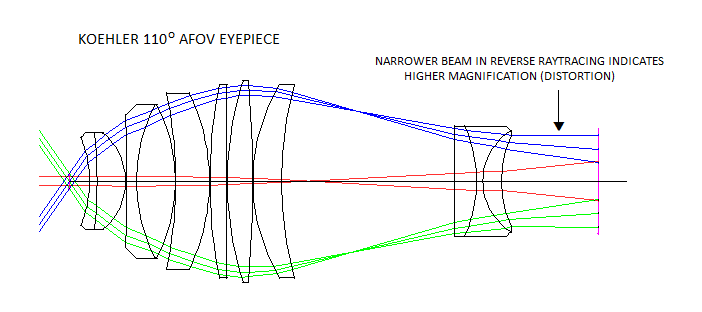telescopeѲptics.net          ▪▪▪▪                                             CONTENTS

# 4.5. IMAGE DISTORTION

As a wavefront aberration, image distortion is caused by the change of magnification with the incident angle, with the actual wavefront being formed tilted with respect to a perfect (Gaussian) reference sphere. It is a consequence of the light cones for oblique angles coming in at a different angles, and using different portions of optical surface(s) than the near-axis cones. It changes the effective focal length for those converging cones, and with it their magnification, i.e. tilt angle. Some surfaces make focal length longer toward the edge, some shorter, and the final sum is the system distortion. In effect, the actual wavefront is tilted with respect to the Gaussian reference sphere, and the actual image point is shifted in the image space. The magnitude of shift increases with the third power of the incident angle, effectively inducing a varying point height magnification in the image space. The result is distortion of the image's geometric form but, since the wavefront remains spherical - or aberrated as determined by other factors - point-image quality itself is not affected. The aberration function of distortion is given by:

Wt = Gρcosθ           (27)

with G=gdα3 being the peak distortion aberration coefficient, g being the aberration coefficient (α is the field angle and d the aperture radius), and θ is the pupil angle. Since ray aberration caused by distortion is independent of pupil coordinates (ρ,θ) all rays meet at the image point, which is displaced radially in proportion to the cube of the point field angle α (FIG. 59).FIGURE 59: Illustration of the effect of image distortion: Gaussian image of a square centered in the field has its corner point C farther away from field center O than its mid-side point M by a factor of 21/2. Since distortion increases with the third power of off-center distance (strictly talking, field angle, but for small angles the difference between the rate of change of the two is negligible) in the image plane the corner point  C is shifted away from its perfect coordinates by a factor (21/2)3 more radially than the mid-side point M (this proportion remain constant, only the magnitude of deformation changes). In other words, the length of the aberrated extension CC' or CC" of the aberrated image of the square is larger than MM' or MM", respectively, by a factor of (OC/OM)3. As a result, the image is deformed, either inward (negative, or barrel distortion), or outward (positive, or pincushion distortion). Since the amount of shift from the perfect coordinate is in proportion to the cube of off-axis angle, α3, linear distortion of any non-circular form centered in the field increases with the cube of its linear diameter; in effect, shape distortion increases with α2.

Aberration coefficient of distortion for a single surface, refractive or reflective, with the stop at the surface, is given by:with the peak aberration coefficient,

G = gα3D/2            (27.2)

representing the peak wavefront error of tilt with respect to the reference sphere centered at Gaussian focus along the axis of aberration (n and n' are the refractive index of incident and refractive/reflective medium, respectively, and D is the aperture diameter).

The aberration coefficient is zero for both, concave mirror (n=1, n'=-1) and a thin lens with the aperture stop at the surface. Distortion is introduced if the stop is displaced, which means that it is present in multi-element systems with the elements at more than insignificant separation. An exception is a sphere with the stop at its center of curvature, when it also has zero distortion, due to its unique symmetry.

In general, distortion is negligible for small angular images, such are those of telescope objectives. However, it becomes significant at large angles, characteristic of the images viewed through telescope eyepieces. Picture below shows raytrace cross section of a 110-degree AFOV eyepiece, with about 50% positive (pincushion) distortion (entering cones are not near orthogonal to the Smyth lens because this particular eyepiece was designed for a binocular objective).The cones entering (actually exiting, since it is reverse raytracing) eyepiece on the right are different in their width, with the field edge cones being noticeably narrower. That is consequence of their increased magnification; in actual use, the entering cones would be of identical width, and the edge cone's exit pupil smaller, according to their increased magnification. Note thet distortion in reverse raytracing has opposite sign to that in actual use.